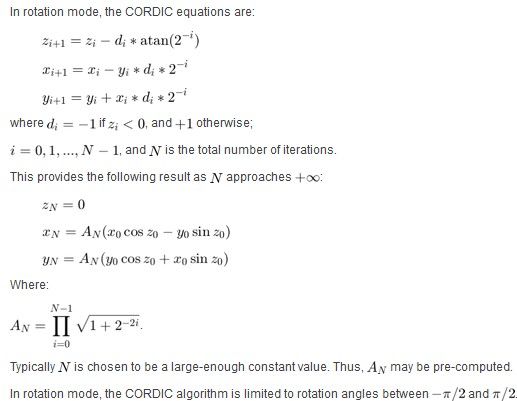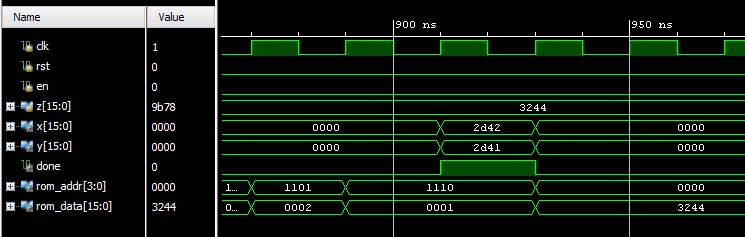# Use Cordic to Calculate Sin/Cos with Verilog Implementation

Make it to the Right and Larger Audience

Blog

# Use Cordic to Calculate Sin/Cos with Verilog Implementation

Here we use Cordic algorithm to calculate cos and sin of an arbitrary angle. The background Cordic algorithm is well described in below Mathworks tutorial:
Compute sine and cosine using cordic rotation kernelSo to us, z0 is the arbitrary angle. To calculate its sine and cosine values, we need to set x0 to be 1/A_N and y0 to be 0. Then cordic outputs will be just x_N = cos(z0) and y_N = sin(z0).

In this algorithm, atan(2^(-i)) may look complicated to calculate. The key is it can be pre-calculated and stored as constant in memory.

The matlab code to calculate x0 and atan(2^(-i)) are as below.

Here is the result after running “cordic” in Matlab:

atan_hex is the pre-calculated atan(2^(-i)) assuming 16bit signed integer. For example, atan(2^0) = 0x3244, atan(2^(-1))=0x1DAC, and so on. x0 is calculated as 0x26DD. Four testAngles selected to test are pi/2, pi/4, pi/8, and 0. Their hex value are 0x6488, 0x3244, 0x1922, and 0x0 correspondingly. Cosine and sine results are also shown. Here again we use 16bit signed integer.

The Verilog code with testbench is also provided. They are based on other’s work online. Some modifications are made to make it work on my side. Full Verilog code with above m-code are in attached zip file. Here we give part of cordic implementation:

Simulation result is as below:So when done=1, x and y outputs are the calculated cosine and sine correspondingly. The following are summary of results.

 angle pi/2 pi/4 pi/8 0 -pi/8 -pi/4 -pi/2 cos/x_N 0002 2d42 3b1f 3fff 3b23 2d43 ffff sin/y_N 3fff 2d41 1880 0004 e785 d2be bfff

Comparing above table with m-code result, we can see results are very close.

Here is the full Verilog implementation with testbench and m-code:Senior Engineer
Author brief is empty
Groups:

Tags:

## cordic

1.hossein 4 years ago
0
-0

module cordic_core #(parameter DATA_WIDTH = 16, ADDR_WIDTH = 4)
(
input clk, rst, en,
input signed [DATA_WIDTH-1:0] z,q,
output done,
output signed [DATA_WIDTH-1:0] x,y
);
reg [4:0] n,n_next;
reg done_int, done_dly;

reg signed [19:0]x_reg,y_reg,x_next,y_next,z_reg,z_next;

reg [1:0] state,state_next;

parameter idle = 1’b0, iteration = 1’b1;

always @ (posedge clk, posedge rst)
begin
if (rst)
begin
state <= idle;
n <= 0;
x_reg <= 0;
y_reg <= 0;
z_reg <= 0;
done_dly <=1;
end
else
begin
state <= state_next;
n <= n_next;
x_reg <= x_next;
y_reg <= y_next;
z_reg <= z_next;
done_dly >> n);
y_next = y_reg + (x_reg >>> n);
z_next = z_reg – q;
end
else
begin
x_next = x_reg + (y_reg >>> n);
y_next = y_reg – (x_reg >>> n);
z_next = z_reg + q;
end
end
endcase
end

assign done = done_redge;

assign done_redge = done_int & (~done_dly);
assign x = done_redge? x_reg: 0;
assign y = done_redge? y_reg: 0;

endmodule`

0
2.hossein 4 years ago
+2
+2 -0

module cordic_core #(parameter DATA_WIDTH = 16, ADDR_WIDTH = 4)
(
input clk, rst, en,
input signed [DATA_WIDTH-1:0] z,q,
output done,
output signed [DATA_WIDTH-1:0] x,y
);
reg [4:0] n,n_next;
reg done_int, done_dly;

reg signed [19:0]x_reg,y_reg,x_next,y_next,z_reg,z_next;

reg [1:0] state,state_next;

parameter idle = 1’b0, iteration = 1’b1;

always @ (posedge clk, posedge rst)
begin
if (rst)
begin
state <= idle;
n <= 0;
x_reg <= 0;
y_reg <= 0;
z_reg <= 0;
done_dly <=1;
end
else
begin
state <= state_next;
n <= n_next;
x_reg <= x_next;
y_reg <= y_next;
z_reg <= z_next;
done_dly >> n);
y_next = y_reg + (x_reg >>> n);
z_next = z_reg – q;
end
else
begin
x_next = x_reg + (y_reg >>> n);
y_next = y_reg – (x_reg >>> n);
z_next = z_reg + q;
end
end
endcase
end

assign done = done_redge;

assign done_redge = done_int & (~done_dly);
assign x = done_redge? x_reg: 0;
assign y = done_redge? y_reg: 0;

endmodule

0